# 基于概率的矩阵分解原理详解（PMF）

p(U|σ2U)=Ni=1N(Ui|0,σ2UI) $p(U|\sigma_U^2)=\prod_{i=1}^NN(U_i|0,\sigma_U^2I)$

p(V|σ2V)=Ni=1N(Vi|0,σ2VI) $p(V|\sigma_V^2)=\prod_{i=1}^NN(V_i|0,\sigma_V^2I)$

P(R|U,V,σ2)=Ni=1Mj=1[N(Rij|UTiVj,σ2)]Iij$P(R|U,V,\sigma^2)=\prod_{i=1}^N\prod_{j=1}^M[N(R_{ij}|U_i^TV_j,\sigma^2)]^{I_{ij}}$

P(U,V|R,σ2,σ2U,σ2V)=P(R|U,V,σ2)P(U|σ2U)P(V|σ2V) $P(U,V|R,\sigma^2,\sigma_U^2,\sigma_V^2)=P(R|U,V,\sigma^2)P(U|\sigma_U^2)P(V|\sigma_V^2)$ =Ni=1Mj=1[N(Rij|UTiVj,σ2)]IijNi=1N(Ui|0,σ2UI)Mj=1N(Vj|0,σ2iI) $=\prod_{i=1}^N\prod_{j=1}^M[N(R_{ij}|U_i^TV_j,\sigma^2)]^{I_{ij}}\prod_{i=1}^NN(U_i|0,\sigma_U^2I)\prod_{j=1}^MN(V_j|0,\sigma_i^2I)$

P(x1,x2,,xn)=fD(x1,x2,xn|θ) $P(x_1,x_2,…,x_n)=f_D(x_1,x_2,…，x_n|\theta)$

1. 由总体分布导出样本的联合概率函数 (或联合密度);

2. 把样本联合概率函数(或联合密度)中自变量看成已知常数,而把参数 看作自变量,得到似然函数L( θ $θ$);

3. 求似然函数L(θ$θ$) 的最大值点(常常转化为求ln L( θ $θ$)的最大值点) ，即θ$θ$的MLE;

4. 在最大值点的表达式中, 用样本值代入就得参数的极大似然估计值 .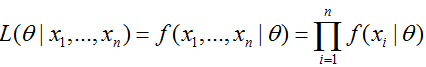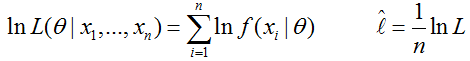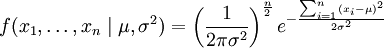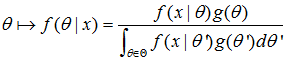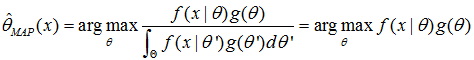RUV $R，U，V$的联合密度对数化: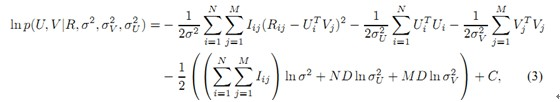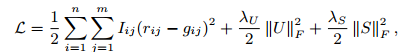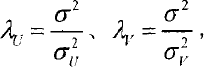PMF也有改进的地方，它没有考虑到评分过程中用户的个人信息，比如有的用户就是喜欢打低分，有的项目（电影）质量就是不高，分肯定高不了等，这样可以采用加入偏置的概率矩阵分解（贝叶斯概率矩阵分解BPMF），将在后面的博客中写出，会给出链接。12-03
04-28
02-21
04-29
12-09657
07-054467
03-162万+
09-141万+
05-30
10-131万+
01-273万+
07-242745
07-192万+
07-221万+
05-30838
10-16
©️2020 CSDN 皮肤主题: 大白 设计师:CSDN官方博客